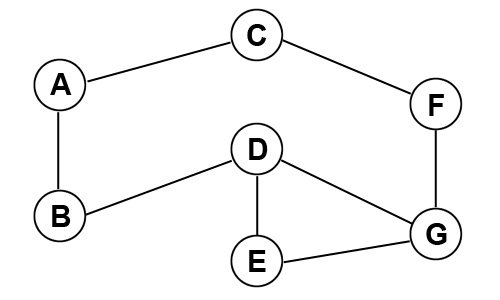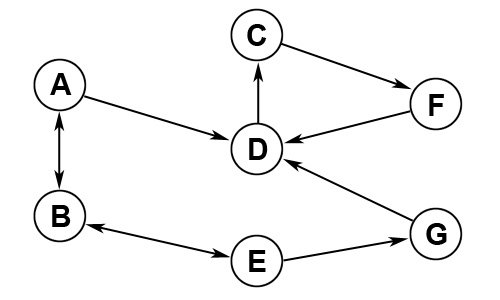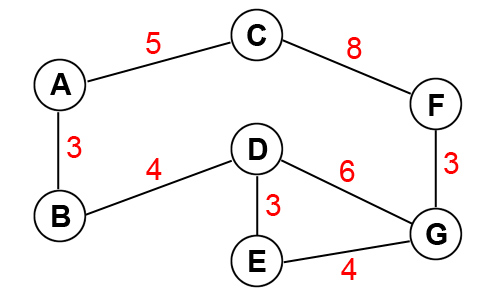Java Chapter 18 - Graphs

18.1 Graphs

A graph is a data structure that can be used for finding paths shortest paths in real world problems.  A graph consists of vertices (nodes) and edges (lines).

1.  Undirected Graph - The graph below has vertices 7 vertices (A - G) and 8 edges connecting them.  There are 3 different paths to travel from A to G.2.  Directed Graph - This graph has directed edges that allow you to only travel between vertices in the direction of the arrow.  There is only 1 path to travel from A to G - (A:B), (B:E), (E:G)3.  Weighted Graph - This graph has a weight for each edge.  This could be used for calculating distances between objects.  Using the weights, we see the shortest path from A to G is 13 - (A:B), (B:D), (D:G)18.2 JGraphT Library

- TextPad: Go to Configure, Preferences, Environment Variables.  Add a path to your jar to the CLASSPATH:
C:\java\jgrapht-0.9.0\lib\jgrapht-core-0.9.0.jar;%CLASSPATH%

- Eclipse: Go to the properties of your project, select Java Build Path, Libraries tab, and Add JAR.

Below are some of the methods in the graph library.

 Creating a Graph Description DirectedGraph g =   new DefaultDirectedGraph     (DefaultEdge.class); Create a directed graph of Strings named g SimpleWeightedGraph g =   new SimpleWeightedGraph   (DefaultWeightedEdge.class); Create a weighted graph of Strings named g Methods Description g.addVertex("Dallas"); Adds a vertex named Dallas g.containsVertex("Dallas"); Returns true if Dallas exists g.addEdge("Dallas","Austin"); Adds an edge to the graph g.setEdgeWeight(g.getEdge("Dallas","Austin"),200); Set edge weight between Dallas and Austin to 200 g.edgesOf("Dallas") Returns a set of edges touching Dallas g.vertexSet() Returns a list of all vertices g.vertexSet().size() Returns the number of vertices g.edgeSet() Returns a list of all edges g.edgeSet().size() Returns the number edges g.getEdgeWeight(g.getEdge("Dallas","Austin")) Returns the edge weight from Dallas to Austin g.removeVertex("Dallas") Removes vertex Dallas g.removeEdge(g.getEdge("Dallas","Austin")) Removes the edge from Dallas to Austin

The program below creates a directed graph, adds 3 vertices, and 5 edges.  It then finds the shorted path from v3 to v1.